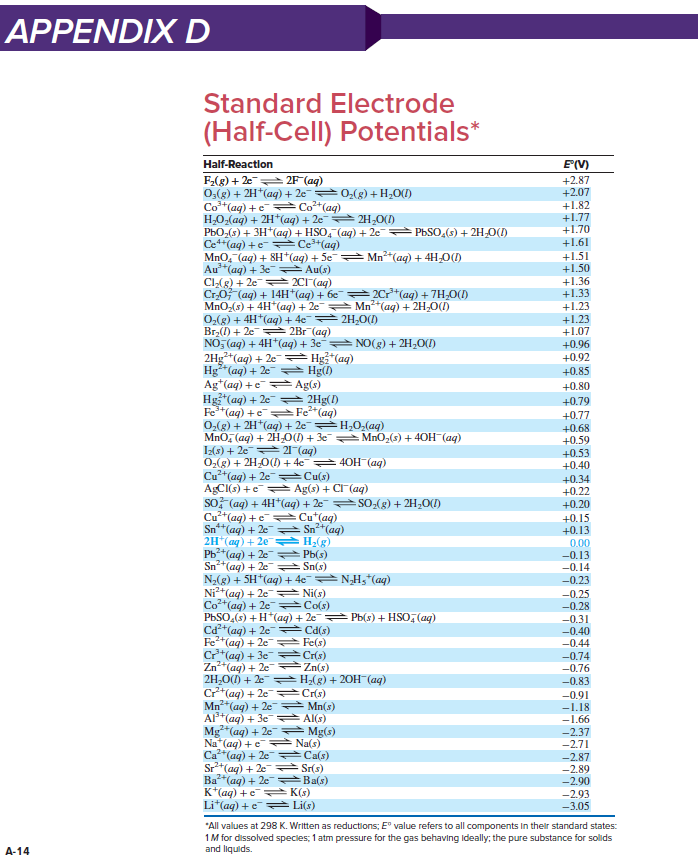# Problem: Balance each skeleton reaction, use Appendix D to calculate E°cell, and state whether the reaction is spontaneous:(b) AgCl(s) + NO(g) ⟶ Ag(s) + Cl−(aq) + NO3−(aq) [acidic]

###### FREE Expert Solution

We are asked to balance the redox reaction under acidic conditions: AgCl(s) + NO(g) ⟶ Ag(s) + Cl(aq) + NO3(aq)

*Before balancing the redox reaction:

ignore H+, OH-, and H2O in the starting reaction

Step 1: Separate the whole reaction into half-reaction

AgCl(s) →  Ag(s) + Cl(aq)                                                 NO(g)→ NO3(aq)

Step 2: Balance the non-hydrogen and non-oxygen elements first

AgCl(s) →  Ag(s) + Cl(aq)                                                 NO(g)→ NO3(aq)

Step 3: Balance oxygen by adding H2O(l) to the side that needs oxygen (1 O: 1 H2O)

AgCl(s) →  Ag(s) + Cl(aq)                                               NO(g) +2 H2O (aq)→ NO3(aq)

Step 4: Balance hydrogen by adding H+ to the side that needs hydrogen (1 H: 1 H+)

AgCl(s) →  Ag(s) + Cl(aq)                                               NO(g) +2 H2O (aq)→ NO3(aq) + 4 H+(aq)

Step 5: Balance the charges: add electrons to the more positive side (or less negative side)

AgCl(s) +1 e-→  Ag(s) + Cl(aq)                                 NO(g) +2 H2O (aq)→ NO3(aq) + 4 H+(aq) + 3 e-

Step 6. Balance electrons on the two half-reactions

▪ multiply the reactions so both half-reactions have the same number of electrons

▪ electrons should cancel in the overall reaction

[ AgCl(s) +1 e-→  Ag(s) + Cl(aq)   ]   x 3

NO(g) +2 H2O (aq)→ NO3(aq) + 4 H+(aq) + 3 e-

Step 7. Get the overall reaction by adding the two reaction.

species that appear in both reactions on opposite sides are subtracted

84% (389 ratings)###### Problem Details

Balance each skeleton reaction, use Appendix D to calculate E°cell, and state whether the reaction is spontaneous:

(b) AgCl(s) + NO(g) ⟶ Ag(s) + Cl(aq) + NO3(aq) [acidic]Frequently Asked Questions

What scientific concept do you need to know in order to solve this problem?

Our tutors have indicated that to solve this problem you will need to apply the Cell Potential concept. If you need more Cell Potential practice, you can also practice Cell Potential practice problems.

What professor is this problem relevant for?

Based on our data, we think this problem is relevant for Professor Maxwell's class at UCF.

What textbook is this problem found in?

Our data indicates that this problem or a close variation was asked in Chemistry: The Molecular Nature of Matter and Change - Silberberg 8th Edition. You can also practice Chemistry: The Molecular Nature of Matter and Change - Silberberg 8th Edition practice problems.